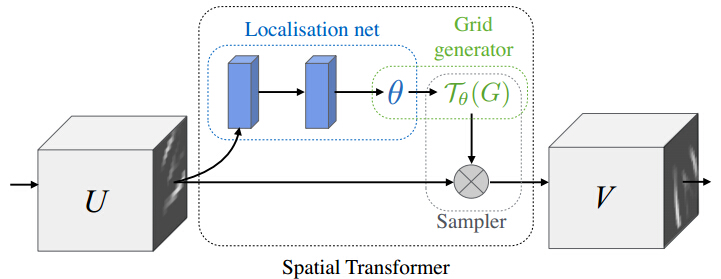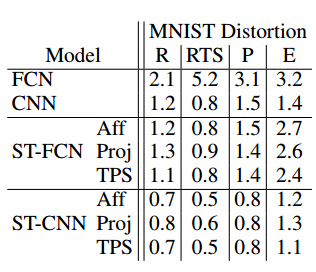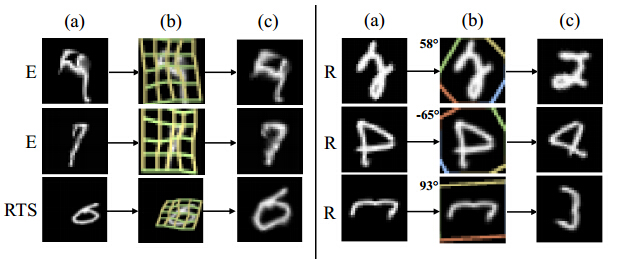# 【论文笔记】Spatial Transformer Networks

——————

### 算法介绍

#### 1. 算法总流程

STN 主要可以分为三个部分：1）localisation network. 2) grid generator. 3) sampler. (中文我翻译不准确，大家意会下)。localisation network用来计算空间变换的参数 θ$\theta$，grid generator则是得到input map URH×W×C$U \in R^{H\times W\times C}$到 output map 各位置的VRH×W×C$V \in R^{H'\times W'\times C}$对应关系 Tθ$\mathcal T_\theta$， sampler根据input map U$U$ 和 对应关系 Tθ$\mathcal T_\theta$，生成最终的output map. 流程图如图所示：#### 1.2 Parameterised Sampling Grid

(xsiysi)=Tθ(Gi)=Aθ(xtiyti)

#### 1.3 Differentiable Image Sampling

Vci=nHmWUcnmmax(0,1|xsim)|max(0,1|ysin|)

VciUcnm=nHmWmax(0,1|xsim)|max(0,1|ysin|)

Vcixsi=nHmWUcnmmax(0,1|xsim)|max(0,1|ysin|)0,1,1if |mxsi|1if mxsiif m<xsi

Vciysi$\frac{\partial{V_i^c}}{\partial{y^s_i}}$Vcixsi$\frac{\partial{V_i^c}}{\partial{x^s_i}}$ 类似。对 θ$\theta$ 的求导为：

Vciθ=VcixsixsiθVciysiysiθ

xsiθ$\frac{\partial{x^s_i}}{\partial{\theta}}$, ysiθ$\frac{\partial{y^s_i}}{\partial{\theta}}$ 根据具体的变换函数便可得到。

### 2. 算法分析

STN 计算较快，几乎没有增加原有网络模型的训练时间。由于它能够在训练过程中，学习到与任务相关的空间变换参数，因此能够进一步最小化网络的损失函数。STN 不只可以用在输入的图像层，也可以加入卷积层或者其它层之后。

### 3. 实验结果### 总结

STN 能够在没有标注关键点的情况下，根据任务自己学习图片或特征的空间变换参数，将输入图片或者学习的特征在空间上进行对齐，从而减少物体由于空间中的旋转、平移、尺度、扭曲等几何变换对分类、定位等任务的影响。加入到已有的CNN或者FCN网络，能够提升网络的学习能力。